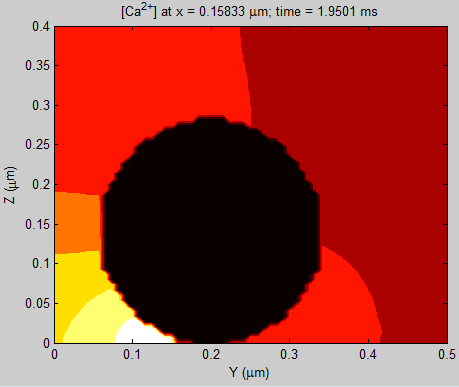Place the two scripts below and the CalC executable in the same directory before running the MATLAB script simple.m. This script calls the CalC script and plots the data produced by CalC. Make sure to use the correct CalC executable name in the first line of the script. Alternatively, you can remove the first line in simple.m, and run simple.par directly using CalC before running simple.m from MATLAB

 ♦ simple.m This MATLAB script executes CalC script `simple.par` below. ♦ simple.par This CalC script simulates Ca2+ from a single open channel close to the location of spherical obstacle representing a vesicle The MATLAB script will produce the following plot (note that [Ca2+] is on log scale):Note that the Windows version of MATLAB has a strange bug where the `system` command may sometimes stop working for no apparent reason; in this case I recommend running MATLAB in command-line mode (`matlab -nosplash -nojvm -nodesktop`), which seems to always solve this problem.

Supported in part by the National Science Foundation grant DMS 0817703 to Victor Matveev

Victor Matveev